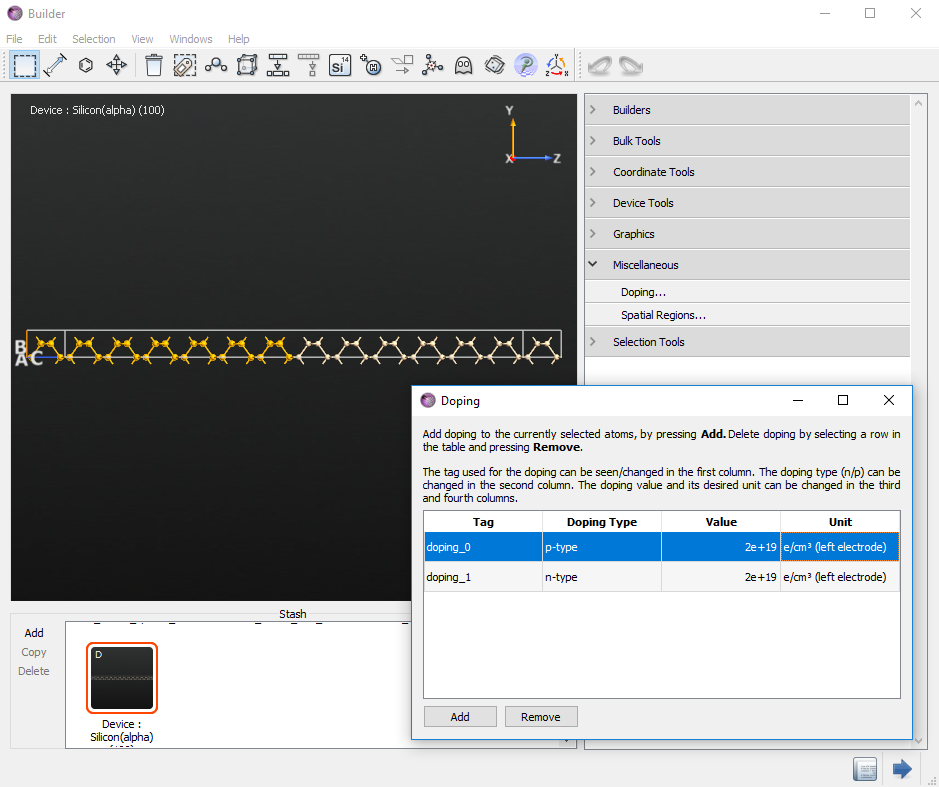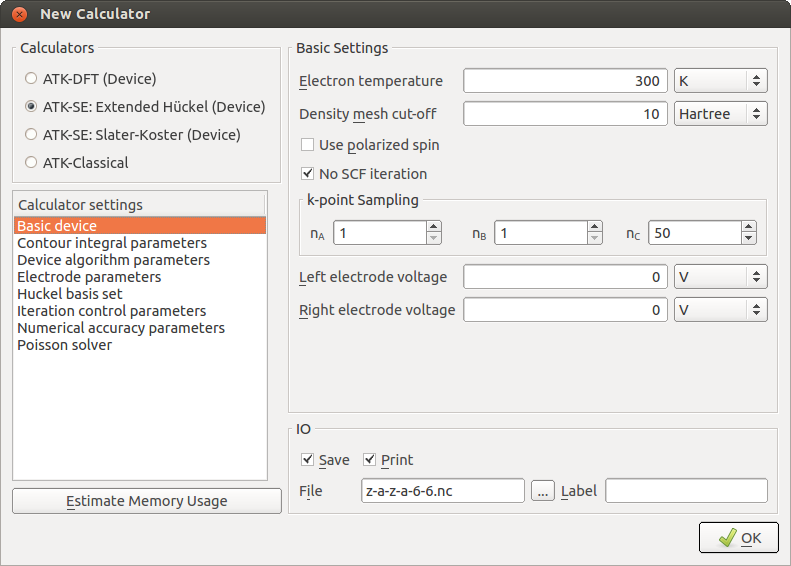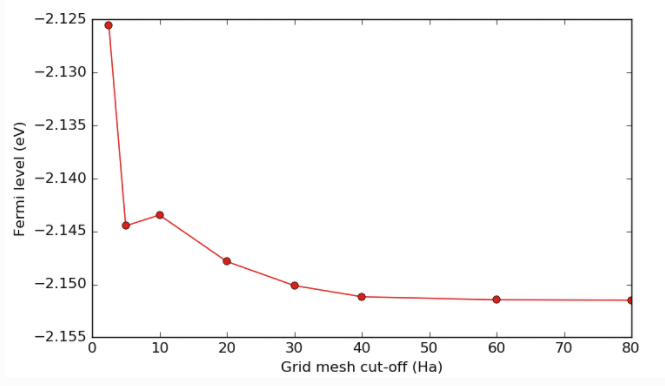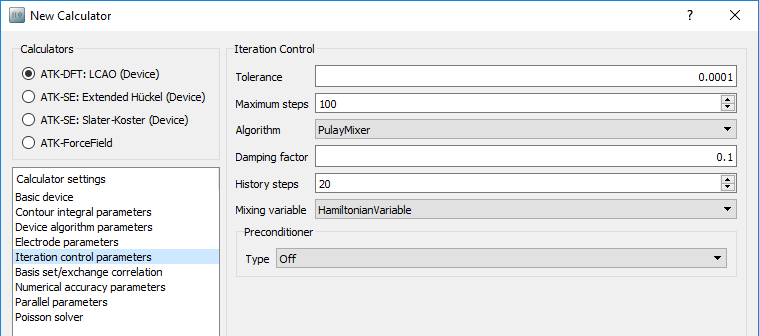# NEGF Convergence Guide¶

Version: 2018

QuantumATK delivers a market-leading and highly optimized implementation of the non-equilibrium Green’s function (NEGF) method for electron transport calculations. Even so, achieving fast and reliable convergence of the NEGF calculation to obtain the electronic ground state can sometimes be challenging. This guide therefore provides a list of action points for troubleshooting poorly converging NEGF calculations.

What does it mean that my calculation did not converge?

Just like in standard calculations for periodic bulks, the NEGF device calculator uses the self-consistent field (SCF) method to iteratively search for the electronic ground state. In each step of the SCF cycle, the electronic structure problem is solved and the total energy is computed. The calculation is converged when the energy change between SCF iterations falls below some tolerance, that is, when the self-consistent electron density is found.

If the calculation does not converge, it means that the self-consistent solution to the electronic structure problem (the ground state) was not found within the allowed number of SCF iterations. Your task is then to modify the NEGF calculation such that it will converge, before moving on to analysis.

Tip

QuantumATK issues a warning if the calculation did not converge
The QuantumATK calculator log will contain the following warning message whenever a calculation did not properly converge:

################################################################################
#                                                                              #
# Warning: The calculation did not converge to the requested tolerance!        #
#                                                                              #
################################################################################


Checking the QuantumATK log for such warnings is an essential habit, as the results are probably not useful if the calculation was not converged. The log stream is sent to the system stdout and/or to a file, depending on how the job is executed. From the O-2018.06 release, the log-file will also contain a Convergence Report with detailed information, if the calculation did not converge.## Introduction¶

As outlined above, it is important that the device calculation converges to the ground state. When this does not happen in a zero-bias calculation, the reason is usually either an improper setup of the device configuration or suboptimal settings in the NEGF device calculator. Finite-bias calculations may sometimes present additional convergence problems that we here address as well.

### Troubleshooting steps¶

The lists below give a troubleshooting guide for both zero-bias and finite-bias calculations.## Zero-bias NEGF calculations¶

### 1. Increase the length of the device central region¶

A very common cause of convergence difficulties is that the central region of the device is too short. Specifically, it is too short for the carriers in the central region to screen the electrode from the effects of the interface. In other words, the NEGF formalism requires that the electrostatic potential is equilibrated and become flat before reaching the electrode. If that is not the fulfilled, the NEGF algorithm will not be able to match it to the bulk-like electrode. In this case the remedy is simple – make the central region longer.

Note

For more information on the importance of screening, please see the page describing the NEGF: Device Calculators.

In the figure below, we show the Hartree Difference Potential for a device with metal on the left and silicon on the right. The vertical line indicates the interface between the two materials.Fig. 203 A plot of the Hartree Difference Potential along the silicon screening region length. Note how 80 Å is not sufficient to allow the potential to become flat.

On the plot we see that the potential is not flat 80 Å of silicon away from the interface, but from 100 Å, it is. On the metal side, we see that just a few Ångstrom are enough to screen the interface and make the potential flat, as expected.

It is possible to decrease the length of the depletion region, i.e. the length of the semiconductor where screening takes place, by adding doping, or if already doped, by increasing the doping level. In the figure below, we show the depletion length vs. the doping level for silicon.Fig. 204 A plot of screening (depletion) length vs. doping concentration. For instance, a doping of 1019 cm-3 corresponds to a depletion length of around 100 Å.

### 2. Increase the length of the electrodes¶

Another common cause of convergence issues is the size of the electrodes. It is very important that the electrodes are long enough to ensure that there is no direct interaction between second-nearest neighboring cells in the transport direction. Phrased another way; there must be no interaction between the electrode extension and the first periodic image of the electrode. A good rule of thumb is that the electrode should be more than twice as long, in the transport direction, as the longest basis function in the basis set. The length of the basis functions can be seen by plotting them in the New Calculator widget.

A more thorough test is to use the Electrode Validator tool in NanoLab. To use it, first do the self-consistent calculation of the electrode, then select the resulting BulkConfiguration on the LabFloor, and click on the Electrode Validator tool in the plugin bar on the right. NanoLab will then inform you whether the electrode is valid, i.e. long enough to fulfill the above criterion.

### 3. Check that the boundary conditions are correct¶

The next item on the list is to make sure that the calculation uses a Poisson solver with the correct boundary conditions. The boundary conditions should be periodic in A and B if the device is to be modeled in that way, and they should be Neumann if there is a gate in that direction. Finally, there should be Dirichlet boundary conditions in the C-direction.

### 4. Increase the number of k-points¶

The next thing to check is the k-point sampling in the transport direction. In order to match the Fermi levels between the electrode and the central region, it is important that the electrode k-point grid is very dense along the C-direction (the transport direction). The default density along the C-direction is 150 (compared to between 2 and 7 otherwise) and it is sometimes necessary to increase it even more. Note that, in most cases, the calculation of the electrodes is an insignificant part of the total NEGF calculation time, so there is in general no need to worry about the performance of this part.

It is also important to check that the number of k-points in the two transverse directions is reasonably converged. If they are too low in a way that leads to unphysical results, this can also hamper convergence. In the figure below, we show an example of how the computed Fermi level may depend on the number of k-points in the transverse directions. In this case, we would expect 1, 2 and possibly 3 k-points to give quite bad results, and possibly have convergence problems, whereas from 5 and up, the results are reasonably converged.Fig. 205 The Fermi level depends on the number of k-points. Here we show the dependence along the non-transport A and B directions.

### 5. Use the EquivalentBulk method for the initial density¶

Next option is to try changing the calculation of the initial density to EquivalentBulk, instead of the default NeutralAtom. The default behavior is to create the initial guess for the density as a direct sum of the neutral atom densities. EquivalentBulk does a self-consistent calculation instead, where the central region is treated as a bulk configuration, and uses the resulting density as the initial guess for the NEGF calculation. Note that this calculation can be quite demanding, and may not be as efficiently parallelized over many computing cores as the NEGF calculation itself.

The NeutralAtom method works just fine in most cases, but for some systems it is necessary to use EquivalentBulk to start the device calculation from a better initial guess. However, if your electrodes are not identical and/or you use a Hückel model, it is most likely not an advantage to use EquivalentBulk.

### 6. Increase the electron temperature¶

Particularly in metals or small-gap semiconductors, convergence can be hampered if the states close to the Fermi level are not adequately described. If this is the case, these states will shift between being occupied and unoccupied, leading to convergence problems. This can be mitigated by increasing the temperature (width) for the electron occupation function, and/or trying one of the non-default occupation functions. Note that higher temperatures can lead to slight inaccuracies in the results, as described on the main page about Occupation Methods.

### 7. Improve the accuracy of the contour integral¶

When setting up the calculator in NanoLab, the lower bound of the contour integral is automatically set so low that it encompasses all the valence states in the elements in the configuration. This fact is quite important, and the next thing to do, is to check that this is indeed the case. If you are in doubt, make a DOS calculation of your material to identify the deepest valence states and their energy, and verify that the lower bound of the contour integral is lower in energy than these states.

Sometimes, convergence can be improved by increasing the number of points in the semicircle part of the default SemiCircleContour or switching to the OzakiContour, where the number of poles (equivalent to contour points) can be increased more easily, as there is only one parameter to adjust. Note that each contour point/pole must be calculated separately, like k-points, and the computational cost is thus approximately linear in the number of contour points/poles. For more information, see the manual pages on the NEGF: Device Calculators and the two types of contour integrals: SemiCircleContour and OzakiContour.Fig. 206 Sketch of the contour integral when using the semicircle contour. See the manual page for more details.

### 8. Try a different Poisson solver¶

Convergence can also be helped by changing the Poisson Solver, e.g. from MultigridSolver to ParallelConjugateGradientSolver, as they use different numerical methods. This means the numerical noise in the calculated electrostatics will be different, which can in turn affect convergence in systems with more complicated electrostatics, such as gates. Note also that for larger calculations, which are run in parallel, ParallelConjugateGradientSolver will usually be faster.

### 9. Increase the density mesh cut-off energy¶

The final thing to check for a zero-bias calculation is the density mesh cutoff. As with k-points in the transverse direction, it should be high enough to provide physically correct results. Ensuring that it is properly converged with respect to the quantity of interest will also help with convergence. In the figure, we show how the Fermi level depends on the mesh cutoff, and it is seen how very low mesh cutoffs can give an inaccuracy in the determination of the Fermi level. However, note also the difference in scale between this and the figure with k-points above.Fig. 207 The computed Fermi level depends on the grid mesh cut-off.

## Finite-bias NEGF calculations¶

In general, finite-bias calculations are harder to converge than zero-bias, and all of the points above apply also in this case. However, there is one further thing to try, which will be explained in the next section, and should be tried as the first thing if the zero-bias calculation converged nicely.

### 1. Always restart from a converged calculation¶

A finite-bias calculation should always restart from a converged calculation with a lower, or zero, bias. If it does not converge, it will often help to decrease the bias difference. For instance, if you want to do a 0.4 V calculation starting from zero bias, the best approach is to do it incrementally, e.g. in steps of 0.1 V. This example script will do exactly that: iv.py

# Set bias
# Positive: Forward
# Negative: Reverse

bias_list = [0.10, 0.20, 0.30, 0.40]*Volt

zero_bias_file = 'device_zero_bias.hdf5'

for bias in bias_list:
if processIsMaster():
print("Bias is now: ", bias )
# Get the calculator
calculator = device_configuration.calculator()

# Set the bias voltage
calculator=calculator(electrode_voltages=(bias/2, -bias/2))

# Attach the calculator and use the old initial state
device_configuration.setCalculator(
calculator(),
initial_state=device_configuration)
device_configuration.update()
nlsave('device_bias_%.2f.hdf5' % bias.inUnitsOf(Volt), device_configuration)


This approach becomes even more relevant for higher biases, where shorter increments might be needed to achieve convergence. So in the case above, maybe 0.1 and 0.2 V converge, but 0.3 V do not. It would then be recommended to try 0.25 V, starting from 0.2 V and using increments of 0.05 V from there. Whether this approach is practical, of course depends on the system under study and the final bias you wish to achieve.

## SCF iteration control parameters¶

If you have already verified that the physical model employed in your NEGF calculation is sane and appropriate (cf. the preceding sections), but the calculation still fails to converge, tuning the parameters that control the SCF loop is the last option.

The SCF parameters are defined in the IterationControlParameters class in ATK Python – navigate to the Iteration control parameters tab in the New Calculator widget to adjust the settings.### 1. Maximum steps¶

Even a healthy and well-behaved NEGF calculation can sometimes converge slower than expected towards the ground state, and may therefore require extra SCF steps to converge correctly.

• In such cases, simply increase the parameter Maximum steps.
• However, first consult the QuantumATK log file from the failing calculation to check that the SCF loop is actually well-behaved, that is, converging towards a reasonable ground state. If not, additional SCF steps are not likely to solve the problem – consider instead adjusting the Pulay mixing.

#### What is a reasonable ground state?¶

First of all, Band energies and Hamiltonian matrix elements should converge steadily towards constant values. Inspect the ATK log file: Look for the lines reporting after each SCF iteration the band energy E and the differences in energy (dE) and Hamiltonian matrix elements (dH) from the previous SCF iteration. In a terminal this may conveniently be done using the grep command:

user@machine \$ grep dE atk.log
|   0 E = -70.6148 dE =  5.719151e-01 dH =  7.266251e-01                       |
|   1 E = -56.8671 dE =  1.374766e+01 dH =  5.675282e-01                       |
|   2 E = -58.5695 dE =  1.702319e+00 dH =  1.737177e-01                       |
|   3 E = -59.6745 dE =  1.105067e+00 dH =  9.875918e-02                       |
|   4 E = -59.3529 dE =  3.216787e-01 dH =  4.048304e-02                       |
|   5 E = -59.0695 dE =  2.833359e-01 dH =  2.447425e-02                       |
|   6 E = -59.2082 dE =  1.386839e-01 dH =  2.978777e-03                       |
|   7 E = -59.0397 dE =  1.684759e-01 dH =  1.492602e-02                       |
|   8 E =  -59.078 dE =  3.826926e-02 dH =  1.691435e-03                       |
|   9 E =   -59.05 dE =  2.800614e-02 dH =  2.041091e-03                       |
|  10 E = -59.0559 dE =  5.931576e-03 dH =  1.624175e-03                       |
|  11 E = -59.0553 dE =  6.427625e-04 dH =  2.825693e-04                       |
|  12 E = -59.0543 dE =  1.008929e-03 dH =  1.880459e-04                       |
|  13 E = -59.0542 dE =  1.964723e-05 dH =  6.034241e-05                       |

• The energy and Hamiltonian differences dE and dH should decrease steadily towards the specified tolerance (10-4 by default).
• The energy may oscillate in the beginning of the SCF cycle, but should eventually converge to a constant value.

Secondly, Mulliken populations should be physically reasonable. Inspect the Density Matrix Report for the last few SCF iterations in the ATK log file. For each atom, the quantities DM and DD are reported. The former is the trace of the device density matrix (the Mulliken populations) while the latter is the difference between DM and the neutral-atom charge on the atom (as given by the pseudopotential).

• Atoms in the same local environment should have approximately the same occupation.
• Variations should be systematic and physical, e.g., in an oxide we would expect extra electrons on the oxygen atoms and fewer on the more electropositive neighbors.
• Charge should not build up near the electrodes.

In the example below, we see how these points are fulfilled:

+------------------------------------------------------------------------------+
| Density Matrix Report                             DM        DD               |
+------------------------------------------------------------------------------+
|   0  Si   [    1.907 ,    1.100 ,    1.564 ]    3.89764  -0.10236            |
|   1  Ni   [    0.001 ,   -0.000 ,    2.342 ]   18.21499   0.21499            |
|   2  Si   [    0.001 ,    2.201 ,    3.120 ]    3.89379  -0.10621            |
|   3  Si   [    0.001 ,   -0.000 ,    4.675 ]    3.89556  -0.10444            |
|   4  Ni   [    0.001 ,    2.201 ,    5.453 ]   18.21180   0.21180            |
|   5  Si   [    1.907 ,    1.100 ,    6.231 ]    3.89446  -0.10554            |
|   6  Si   [    0.001 ,    2.201 ,    7.787 ]    3.89564  -0.10436            |
|   7  Ni   [    1.907 ,    1.100 ,    8.565 ]   18.21115   0.21115            |

• The Mulliken population is approximately the same for all Ni and all Si atoms, respectively.
• We see that all Si atoms have a slightly negative value of DD, while the Ni atoms have a corresponding positive value of twice the size.
• The atoms close to the electrode boundary (those with lowest indexes) do not show unphysically large Mulliken populations.

### 2. Pulay mixing¶

The SCF loop uses a PulayMixer to generate the electron density in each SCF step, by mixing densities from previous steps into the guess for the current step. The Damping factor parameter controls the fraction of previous density to use in the mixing, and History steps controls the number of previous steps to use densities from.

The default Pulay mixing parameters should in general give a fast and stable SCF convergence, but sometimes a bit of tweaking can be useful:

• Try first to decrease the Damping factor to 0.05 or even 0.01. This should in particular help in case of metallic states at the Fermi level.
• Increasing the number of History steps may slightly improve convergence, but will also increase the memory consumption.

### 3. Tolerance¶

The SCF loop keeps going until the accuracy tolerance or the maximum number of steps is reached. This tolerance is measured on both the total band energy and the Hamiltonian matrix elements – in both cases the absolute difference from one SCF step to the other must be below the specified tolerance in order for the calculation to be considered accurate and converged.

• Increasing the Tolerance above the default value may in rare cases be needed to get the NEGF calculation to converge, but it may also lead to an inaccurate ground state. A high tolerance should therefore only be used as a last resort! In fact, it is more common to tighten the SCF tolerance (lowering it to e.g. 10-6) to improve the accuracy of the converged ground state.

## Contact support¶

If everything fails, write a post on the online QuantumATK Forum (https://forum.quantumatk.com) or contact QuantumATK support (quantumatk-support@synopsys.com). In both cases, please attach your ATK script and log file.